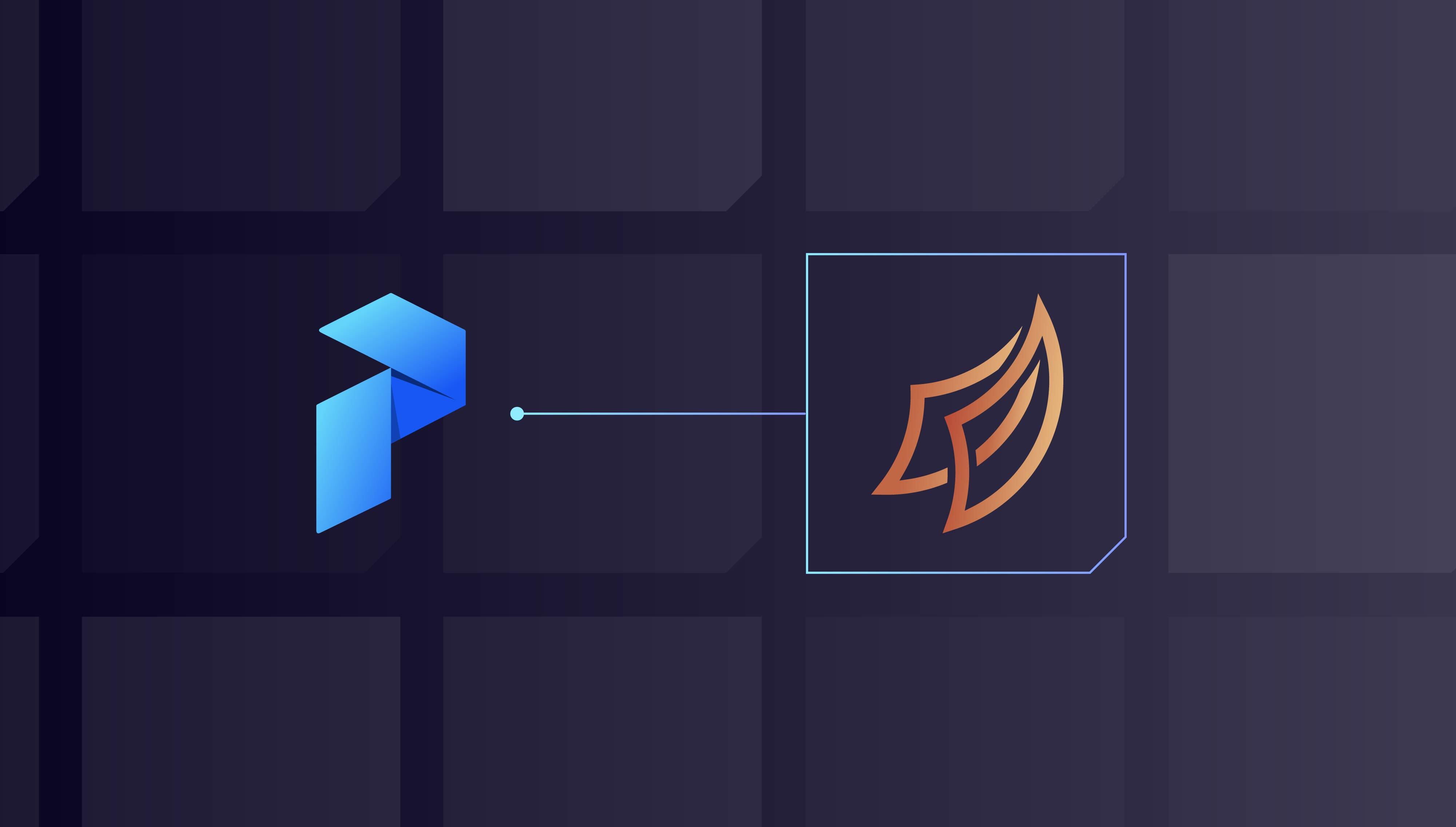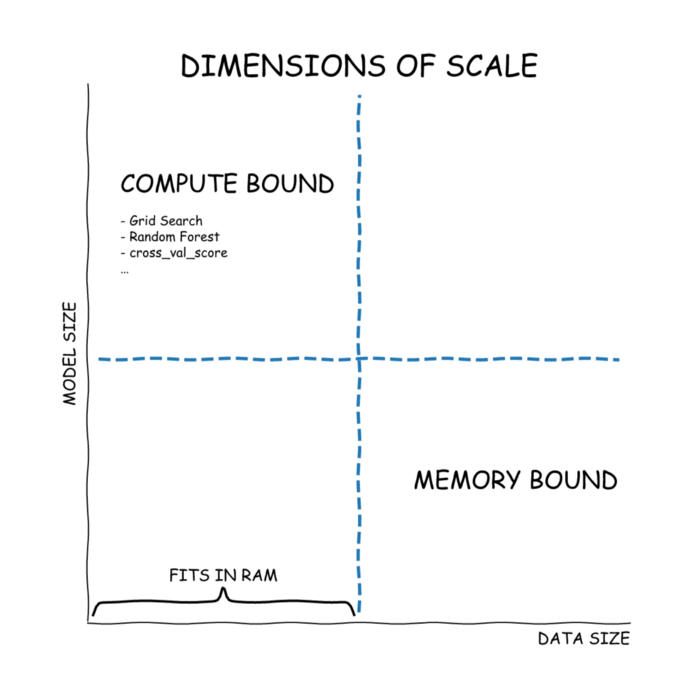###### Feb. 14, 2022

Introducing Prefect-ML: Orchestrate a Distributed Hyperparameter Grid Search on Dask

Are you familiar with the hottest new hyperparameter tuning framework called Prefect-ML? Well probably not, because it doesn’t exist. However, even though Prefect is primarily a workflow orchestration tool, it actually provides us with a lot of building blocks to perform hyperparameter tuning and tracking of machine learning (ML) experiments. By leveraging the built-in DaskExecutor, we can parallelize training ML models distributedly over a Dask cluster. This blog will show how Prefect’s mapping can be used as an interface for hyperparameter training.

This article assumes minimal ML knowledge. The final code can be found in this Github repository.

When working on ML problems, data scientists often have to fine-tune their models to improve performance. Fine-tuning involves trying different combinations of model hyperparameters — the parameters used to govern the model training, making the fitting process more aggressive or less aggressive. The image below from AWS documentation gives us a good intuition for what “more aggressive” or “less aggressive” looks like.

The model on the right side is too aggressive and unlikely to generalize well to new data when put in production. So how do we find this sweet spot? By trying different hyperparameter combinations and looking at the metrics.

One such approach is to create a set of possible models and hyperparameters. For each item in this set, we train the model and look at the metrics generated. This is called a grid search because we make a grid of potential hyperparameter combinations. After collecting the results of a grid search, we can fine-tune the search space and create more informed combinations of training jobs. After enough iterations, we eventually will have converged to a good set of hyperparameters.

Because a grid search is comprised of independent training jobs, we can actually save time by running these jobs in parallel on all available cores of our machine. When our machine isn’t powerful enough, we can utilize a distributed cluster to run multiple jobs in parallel.

Before we dive into code, it’s important to distinguish that there are two forms of distributed machine learning. The dask-ml documentation does a good job of distinguishing the two.

The first type is memory-bound problems. This is when the data doesn’t even fit on a single machine, so one machine learning model is trained across a cluster simultaneously. This requires communication between the different workers during the intermediate steps.

The second type of problem is compute-bound. In this scenario, we want to train potentially hundreds of models that are independent, and a cluster speeds up the execution of these training jobs.dimensions of scale chart illustrating the two types of distributed machine learning: memory-bound, and compute-bound.

Thinking about independent job runs, Prefect, as a workflow orchestrator, already provides an elegant interface around parallelizing independent tasks in the form of mapping. We’ll use this interface to orchestrate our ML training jobs over Dask.

In practice, there are other libraries that are optimized to handle this problem such as ray-tunetune, and dask-ml, but if we’re already using Prefect, it’s very easy to extend what we already know without having to learn a new tool.

As with any ML tutorial, we’ll start by grabbing a dataset. We’ll use the Titanic dataset from Kaggle, a very popular dataset for ML tutorials. We’ll download the `train.csv` and name it `titanic.csv`. This lets us load it with the following code.

For those unfamiliar, the Titanic dataset contains the passengers of the Titanic, and the goal is to predict whether or not they survived using ML. The dataset looks like this:

Kaggle provides a good explanation of each of the columns on the dataset page. For our demo, we’ll just get the simplest columns to train a model on.

For the grid search later, we will try a couple of different models. Some of them can’t handle NULL values, and some of them can’t handle categorical features (variables), so we need to massage the data a bit with the code snippet below. Here, we drop the useless columns, turn `Sex` from `male` and `female` to 1 and 0, and one-hot encode the `Embarked` column with the `pandas.get_dummies()` function.

For those unfamiliar, one-hot encoding is replacing one column with N categories to N binary columns that contain 1 or 0. This helps machine learning models work with numbers rather than categories.

The new data will look as follows (the last three columns are the one-hot-encoded `Cabin` column):

The point here is that we have a purely numeric dataset, which is a requirement for some models.

Now that we finished preparing the data, we need to split it into a training dataset and the testing dataset. Earlier we mentioned that some models don’t generalize well to new data they weren’t trained on. Splitting our dataset allows us to simulate how well our model generalizes because we calculate the performance metrics on the held-out test set.

In the code snippet below, `X` is the data we are using to make a prediction and `y` is the thing we are predicting (Survival). After we split the dataset, we fill the NULL `Age` values with the mean `Age` of the training dataset. It is a best practice to calculate this mean from the training dataset only because we “don’t know” the test dataset records ahead of time.

Here is where the fun begins. We can create a `train_model()` function to train a model. It’s a generic wrapper that takes in the model and data. In order, it will:

• fit the model on the training data

• create predictions from the test set

• compare the predictions from the real test set values

• return the model used and the accuracy values

We can test this function with a `LogisticRegression()` model, producing the output shown below. The important part is that it contains the model name, accuracy, and the parameters that went into the model (all these are default values), which are necessary to reconstruct the model.

Because of the uniform `scikit-learn` interface, all of the available models have `.fit()` and `.predict()` methods. This makes our `train_model()` function work on any of the `scikit-learn` classifiers. Here we pass a different classifier to our function. This one has a much lower accuracy (68.72%).

The code written so far will serve as the backbone of distributing these jobs.

To bring our code to Prefect, we just need to wrap our previously written code into functions decorated by the `@task` decorator. This `create_data()` task is just the code we had earlier wrapped in a function. `nout=4` in the `@task` decorator means there are four outputs of the task.

The code block below lists the models we will be using for our machine learning training jobs. There are five different types of models we will try. For the, we will try two sets of hyperparameters. We could keep going and generating parameter combinations as much as we want, but we’ll learn a better way to do it later. The `get_models()` task will return this list.

Next, we have the `train_model()` task. This is exactly the same as before.

Lastly, we make a `get_results()` task that will get all of the training jobs, create a DataFrame from them, and then log the values to show us the results.

Here is where we piece together all the tasks that we created. It should look straightforward except for the `train_model()` call where we `.map()` over the models. Because `get_models()` creates a list of 6 models, `train_model` will map over these six models and run once for each. Because the `X_train``X_test``y_train``y_test` remain the same over the training jobs, we mark them as `unmapped`.

The code will still run sequentially even if we use mapping because we didn’t define the Prefect executor. The default executor is a sequential `LocalExecutor`. For local parallelism, we can attach the `LocalDaskExecutor` to our Flow. Now, this is ready to utilize all the available cores of our machine.

But of course, this article is about distributing over a Dask cluster. In this case, we just need to use the `DaskExecutor` and configure it to spin up a temporary cluster or point to an existing cluster. Prefect will then take care of submitting tasks to the cluster for execution.

```from prefect.executors import DaskExecutor flow.executor = DaskExecutor(insert_configuration_here)```

More information on configuring the `DaskExecutor` can be found in the docs.

One of the elegant things about using the `mapping` interface is that the execution environment is separated out from the logic. We just need to add an executor to go from local execution to distributed execution.

The last part is to register and run with Prefect Cloud and see what the output looks like. If you recall, we logged the results of our hyperparameter tuning experiments. The image below shows the logs. We can see one of the `RandomForestClassifier` models performed the best.

This view is unsatisfying though because the params are hidden. We can easily improve it by modifying our `get_results()` task to create a markdown artifact to be rendered in the UI instead of just logging the results.

Now we can view the table properly in the UI (after registering and running the flow). Because it’s attached to the Flow Run page, we can return to the Prefect UI in the future and see the experiments we previously ran! We can even create plots to compare model performance based on the increase or decrease of certain hyperparameters.

Looking at the initial set of results, our top three models were the `LogisticRegression` model and the two `RandomForestClassifier`s. At this point, we can consider focusing on these models and fine-tuning these specific models. The `tune` library gives us a scalable and elegant way to define the search space of hyperparameters.

There are two approaches we can take to find better hyperparameters. The first is the Grid Search, where we have a predefined grid of hyperparameter combinations. Sometimes though, using a grid approach can limit our search space. We can also use a Random Search instead, which is just a random combination of possible hyperparameter values.

The code snippet below uses the `tune` library to create the search space. For this specific problem, we’ll combine Grid Search and Random Search. We create two distinct search `Space`s for the `LogisticRegression` and for the `RandomForestClassifier``Grid` means that all of the values should be tried. `Rand` means that a random number from the range is taken, and `RandInt` means a random integer is taken.

This code snippet is a list of dictionaries we can use to iterate over. The first four are generated from `space1` and the next four are generated from `space2` . Notice `space2` is comprised of random values.

Using this search space, we can create a new `get_models()` `task`. Notice this expression is very parameterizable. We can use Prefect parameters to control the number of samples, or maybe even a hyperparameter range of acceptable values. Now, we replace our previous `get_models()` function with the new one that uses `Space`.

After registering and running again via Prefect Cloud, we can view the new artifact.

For more information on the `tune` library, the Search Space docs can be found here and a recent PyData talk can be found here.

Note that in order for this approach to work, the models and data need to be serializable by `cloudpickle` in order to be sent to Dask. Fortunately, `pandas DataFrames` and `scikit-learn` models are serializable, which makes this approach applicable to a lot of use cases. It won’t work for deep learning models that are not serializable.

Even though Prefect is a general-purpose orchestrator, it can be used to train machine learning jobs in a distributed fashion. By using the mapping feature, we can leverage Prefect as an interface to submit model training jobs to Dask. Features such as artifacts and parameters provide building blocks to make Prefect an experiment tracker that can be used to monitor ML workflows alongside data engineering workflows in a single UI. For data professionals already using Prefect, the interface shown here is easy to adopt, yet highly scalable.

Happy engineering!

Posted on Feb 14, 2022
Blog Post